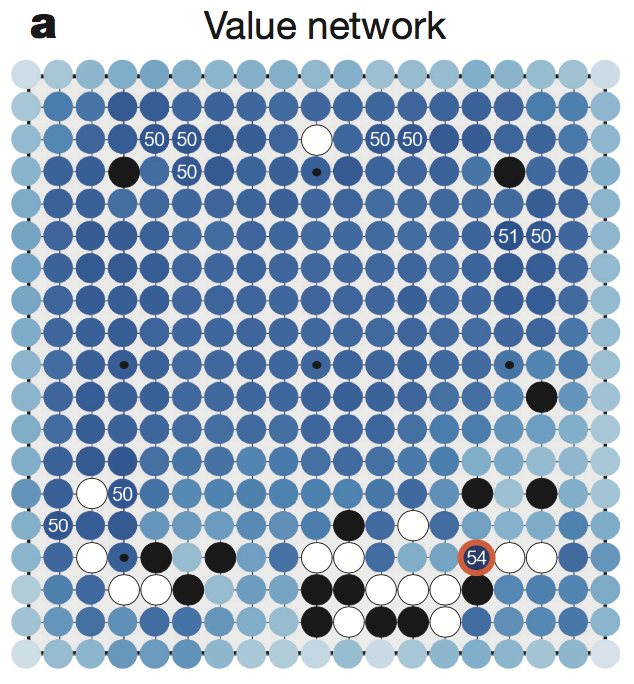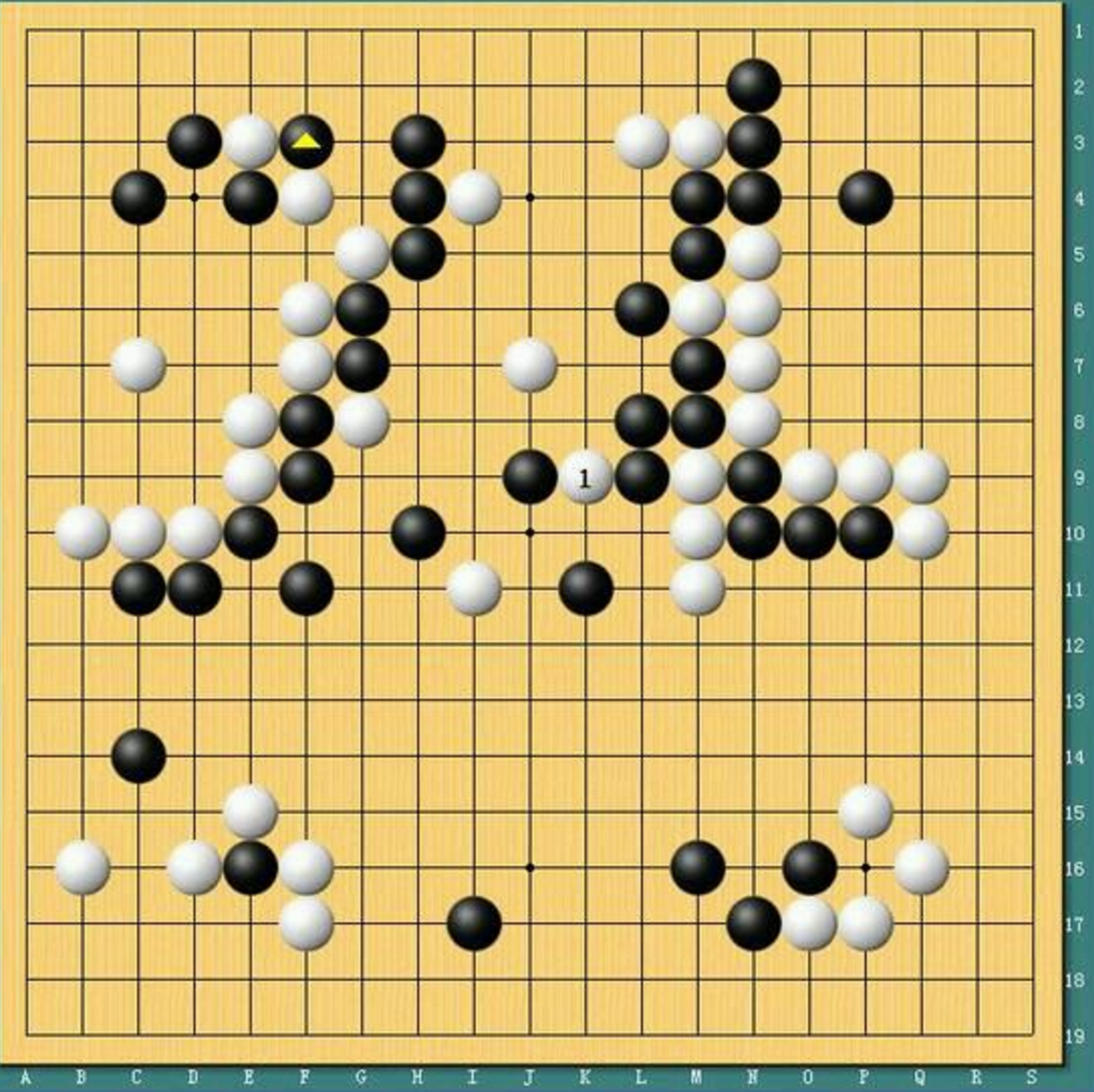【阅读时间】15min 8506 words
【阅读内容】针对论文AlphaGo第一版本，进行了详细的说明和分析，力求用通俗移动的语言让读者明白：AlphaGo是如何下棋的

# 问题分析

$$\vec s = (\underbrace{1,0,-1,\ldots}_{\text{361}})\tag {1-1}$$

$$\vec a = (0,\ldots,0,1,0,\ldots)\tag {1-2}$$

Mastering the game of Go with deep neural networks and tree search

# 问题解决

## 深度卷积神经网络——策略函数（Policy Network）

An Intuitive Explanation of Convolutional Neural Networks （好像原地址挂了）（5.29更新，原地址已经恢复，原地址的排版更好，估计之前那个博主在进行博客的整理）

• 在Deep Learning中，卷积层的中的Filter也需要训练，也就是说我们使用已有数据来学习图像的关键特征，这样，就可以把网络的规模大幅度的降低

### 深度卷积神经网络解决围棋问题

2015年，Aja Huang在ICLR的论文Move Evaluation in Go Using Deep Convolutional Neural Networks中就提出了如何使用CNN来解决围棋问题。

$$\vec a=f(\vec s) \tag{2-1}$$

### 深度卷积网络策略的棋力

Efficient Selectivity and Backup Operators in Monte-Carlo Tree Search

## MCTS 蒙特卡洛搜索树——走子演算（Rollout）

• 面对一个空白棋盘 $\vec s_0$，最初我们对棋盘一无所知，假设所有落子的方法分值都相等，设为1
• 之后，【随机】361种方法中选一种走法 $\vec a_0$，在这一步后，棋盘状态变为 $\vec s_1$。之后假设对方也和自己一样，【随机】走了一步，此时棋盘状态变为 $\vec s_2$
• 重复以上步骤直到 $\vec s_n$并且双方分出胜负，此时便完整的模拟完了一盘棋，我们假设一个变量r，胜利记为1，失败则为0

$$\text{新分数} = \text{初始分数} + r \tag{2-2}$$

$$score(\vec s) = \begin{pmatrix} r_{11} & r_{12} & \cdots & r_{1n} \\ r_{21} & r_{22} & \cdots & r_{2n} \\ \vdots & \vdots & \ddots & \vdots \\ r_{n1} & r_{n2} & \cdots & r_{nn} \end{pmatrix}$$

• 没有任何人工决策的if else逻辑，完全依照规则本身，通过不断的想象（随机）来进行自我对弈，最后提升这一步的质量。有意思的是，其实这也是遵照了人类下棋的思维模式（模仿，只是这一次模仿的不是下棋风格，而是人类思考的方式。十分奇妙，人从飞鸟中受到启发发明了飞机，从鱼身上受到启发发明了潜艇，现在，机器学习的程序，通过学习人类使自身发生进化），人类中，水平越高的棋手，算的棋越多，只是人类对于每一个落子的判断能力更加强大，思考中的棋路，也比随机方式有效的多，但是机器胜在量大，暴力的覆盖到了很多情况。注意，这一个特点也为之后的提高提供了思路
• MCTS可是持续运行。这种算法在对手思考对策的时候自己也可以思考对策。在对方思考落子的过程中，MCTS也可以继续进行演算，在对面落子后，在用现在棋盘的情况进行演算，并且之前计算的结果一定可以用在现在情况中，因为对手的下的这步棋，很可能也在之前演算的高分落子选择内。这一点十分像人类
• MCTS是完全可并行的算法

Aja Huang很快意识到这种方法的缺陷在哪里：初始策略（或者说随机的落子方式）太过简单。就如同上面第一条特点所说，人类对每种 $\vec s$ （棋型）都要更强的判断能力，那么我们是否可以用 $P_{human}(\vec s)$ 来代替随机呢？

Aja Huang改进了MCTS，每一步不使用随机，而是现根据 $P_{human}(\vec s)​$ 计算得到 $\vec a​$ 可能的概率分布，以这儿概率为准来挑选下一个 $\vec a​$。一次棋局下完之后，新分数按照下面的方式来更新
$$\text{新分数} = \text{调整后的初始分} + \text{通过模拟的赢棋概率} \tag{2-3}$$

$$\text{调整后的初始分} = \frac{P_{human}(\vec s)}{(\text{被随机到的次数} + 1)} \tag{2-4}$$

2015年2月，Aja Huang在Deepmind的同事在顶级学术期刊nature上发表的文章 Human-level control through deep reinforcement learning 用神经网络打游戏。这篇文章，给AlphaGo提供的了新的方向

## 强化学习——局面函数（Value Network）

### 什么是强化学习

• 状态 (States) = 环境，例如迷宫的每一格是一个 state
• 动作 (Actions) = 在每个状态下，有什么行动是容许的
• 奖励 (Rewards) = 进入每个状态时，能带来正面或负面的 价值 (utility)

• 方案 (Policy) = 在每个状态下，你会选择哪个行动？也是一个函数

### 自对弈

• 先用 $P_{human}(\vec s)$ 和 $P_{human}(\vec s)$ 对弈，比如1万盘，得到1万个新棋谱，加入到训练集中，训练出 $P_{human-1}(\vec s)$ 。
• 使用$P_{human-1}(\vec s)$和$P_{human-1}(\vec s)$对弈，得到另1万个新棋谱，加入训练集，训练出$P_{human-2}(\vec s)$。
• 同理，进行多次的类似训练，训练出$P_{human-n}(\vec s)$，给最后的新策略命名为$P_{human-plus}(\vec s)$

（感觉一下，这个$P_{human-plus}(\vec s)$ 应该挺强力的！这里回顾一下$P_{human}(\vec s)$是什么：是一个函数，$\vec a=f(\vec s)$ 可以计算出当前 $\vec s$ 下的落子 $\vec a$ 的分布概率）

Aja Huang认为是$P_{human-plus}(\vec s)$走棋的路数太集中，而MCTS需要更加发散的选择才能有更好的效果。

### 计算局部评价函数（Value Network）

• 开局先用$P_{human}(\vec s)$走L步，有利于生成更多局面
• 即使如此，Aja Huang还是觉得局面不够多样，为了进一步扩大搜索空间，在L+1步时，完全随机一个 $\vec a$ 落子，记下这个状态 $v(\vec s_{L+1})$
• 之后使用$P_{human-plus}(\vec s)$来进行对弈，直到结束时获得结果r，如此不断对弈，由于L也是一个随机数，我们可以得到，开局、中盘、官子等不同阶段的很多局面 $\vec s$，和这些局面对应的结果r
• 有了这些训练样本 $\langle \vec s,r\rangle$，还是使用神经网络，把最后一层改成回归而非分类（这里不是用的分类，而是用的回归，拟合），就得到了一个 $v(\vec s)$ 来输出赢棋的概率$v(\vec s)$ 局部评估函数拥有在线下不断自我进化的能力（这也是AlphaGo可以随时间越来越强的最重要的部分）

• $P_{human}(\vec s)$ 我的老师是人类！
• MCTS 乱下，我只看输赢
• $v(\vec s)$ 我能判断局势

## AlphaGo

Aja Huang使用MCTS框架融合局面评估函数 $v(\vec s)$ 的策略是：

• 使用$P_{human}(\vec s)$作为初始分开局，每局选择分数最高的方案落子
• 到第L步后，改用$P_{human-fast}(\vec s)$把剩下的棋局走完，同时调用 $v(\vec s_L)$，评估局面的获胜概率，按照如下规则更新整个树的分数

$$\text{新分数} = \text{调整后的初始分} + 0.5*\text{通过模拟得到的赢棋概率} + 0.5*\text{局面评估分} \tag {3-1}$$

• 前两项和原来一样

• 如果待更新的节点就是叶子节点，局面评估分就是 $v(\vec s_L)$
• 如果是待更新的节点是上级节点，局面评估分是该叶子节点 $v(\vec s)$ 的平均值

### 详解AlphaGo VS 樊麾 对局走下某一步的计算过程

a图使用局部评估函数计算出 $\vec s$ 状态下其他落子点的胜率

b图MCTS中使用局部评估函数加 $P_{human}(\vec s)$ 得出的结果

c图MCTS中使用$P_{human}(\vec s)$（复合算法）和$P_{human-fast}(\vec s)$走子走到底的结果

d图深度卷积神经网络使用策略函数计算出来的结果

e图使用公式3-1和相关流程计算出的落子概率

f图演示了AlphaGo和樊麾对弈的计算过程，AlphaGo执黑，樊麾执白。红圈是AlphaGo实际落子额地方。1，2，3和后面的数字表示他想象中的之后樊麾下一步落子的地方。白色方框是樊麾的实际落子。在复盘时，樊麾认为1的走法更好（这说明在樊麾落子后AlphaGo也在进行计算）

# 总结

AlphaGo的MCTS属于启发式搜索算法

## 李世石 VS AlphaGo 1.0——第四局78手挖打劫围棋术语，一方制造事端，和另一方讨价还价的行为。
劫材可以用来做价格谈判的筹码。通常是走一手没戏，但对手若不予置理，再走第二手会出棋的局部。
寻劫通过目数计算，寻找一些有价值的局部制造事端强迫对手应答。通常价值至少需要和打劫的地方相当或者小不太多，否则对方很容易消劫。
利用劫劫胜可杀死对方或者得到利益，劫败也应该让对方付出代价，除非双方劫材大小和数量相差悬殊。

## AlphaGo 2.0 VS 柯洁——虽败犹荣

• 臆想一，是否可以利用AlphaGo来判断规则是否公平（中国和韩日规则的不同，7目半和6目半）。
• 臆想二，最终AlphaGo的自对弈是接近和棋。可惜AlphaGo已经退役。希望针对Deepmind放出的50盘自对弈棋谱可以研究出一些门道，使得围棋这门竞技本身有更大的突破。

• 一是因为AlphaGo每次MCTS计算都会计算到接近分出胜负，具有前瞻性
• 二是因为局面函数本身就是为了来统计大局形势定义的，具有判断局面优劣的能力

[Reference]